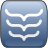## Complex number PHP class

### Description

This is a lightweight, easy-to-use complex number class in PHP. It contains methods representing the basic operations, such as addition, subtraction, multiplication, exponentiation and square root calculation, and also a few complex number specific methods (polar form, conjugate etc.).

### Motivation

As I described here I had to solve quartic equations to overcome a geometric problem in my StellaSphaera project, which wouldn't be possible without a complex number class. So first I tried the PEAR library but for me it was too cumbersome and verbose. As I couldn't find any better solution, I decided to create my own complex number class. Because PHP doesn't support operator overloading it is impossible to deal with complex numbers in the same natural way as we do with integers and floats. However I tried to reduce the roundabouts, so that it is easy to chain operations to create compact, one-line expressions. In addition methods both accept complex and real numbers (integer, float) as arguments, so you don't need to always create a new complex object to pass a real number to a method as with the PEAR class. I only implemented a few operations, but in most cases I think this set is enough or being an open source library it can be easily extended.

### Reference

teComplex
Class representing a complex number.
Fields:
publ float r : Real component.
publ float i : Imaginary component.
Methods:
toString()
Generates a string representation of the complex number contained in the object.
Return value
string String representation of the complex number.
equals(teComplex/float num)
Checks whether the complex number equals to the passed argument.
Arguments
teComplex/float num : Number that the original complex number tested against.
Return value
bool Returns true if the original and the passed number are equal, false if they aren't equal.
abs()
Returns the absolute value (modulus, magnitude) of the complex number, or in other words the length of the vector representing the complex number on the complex plane. Thus the returned value is one of the components of the polar form.
Return value
teComplex The absolute value of the complex number.
arg()
Returns the argument (phase) of the complex number, or in other words the angle of the vector - representing the complex number - with the positive real axis on the complex plane. Thus the returned value is one of the components of the polar form.
Return value
teComplex The argument of the complex number.
neg()
Returns the negative (additive inverse, opposite) of the complex number by negating both the real and the imaginary component.
Return value
teComplex The negative of the complex number.
conj()
Returns the conjugate of the complex number by negating only the imaginary component.
Return value
teComplex The conjugate of the complex number.
inverse()
Returns the inverse (multiplicative inverse, resciprocal) of the complex number by raising it to minus first power.
Return value
teComplex The inverse of the complex number.
Notes
The complex number should be nonzero, or a 'Division by zero while inverting zero' exception is thrown.
Calculates the sum of the original complex number and the passed argument.
Arguments
teComplex/float num : Second term of addition, which can be both complex and real number.
Return value
teComplex The sum of the original number and the num argument.
sub(teComplex/float num)
Subtracts the passed argument from the original complex number.
Arguments
teComplex/float num : The subtrahend, which can be both complex and real number.
Return value
teComplex The difference between the original number and the num argument.
mul(teComplex/float num)
Multiplies the original complex number by the passed argument.
Arguments
teComplex/float num : The multiplier, which can be both complex and real number.
Return value
teComplex The product of the original number and the num argument.
div(teComplex/float num)
Divides the original complex number with the passed argument.
Arguments
teComplex/float num : The divisor (denominator), which can be both complex and real number.
Return value
teComplex The quotient of the original number and the num argument.
Notes
The passed number should be nonzero, or a 'Division by zero' exception is thrown.
pow(teComplex/float num)
Exponentiation. Raises the original complex number to the specified power.
Arguments
teComplex/float num : The exponent, which can be both complex and real number.
Return value
teComplex The original number as base raised to the power of the num argument.
Notes
If the exponent is a fraction the principal root is returned.
sqrt()
Calculates the square root of the original complex number.
Return value
teComplex The square root of the original number.

### Live demo

Coming soon!

 Repo: Ferrari PHP Date: 2013.05.13. License: MIT License Language: PHP Author: Bence Ágg